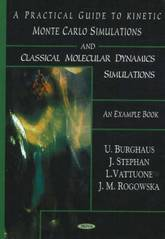Filter
(found 547 products)The guidelines of this textbook are numerous example programs, flux diagrams, schemes, and figures presenting the obtained results. Step by step, the authors explain how steady state Monte Carlo Simulation (MCS) and time resolved, so-called kinetic or dynamic Monte Carlo Simulation (KMCS), schemes, respectively, can be set up. Furthermore, examples ...
Practical Guide to Kinetic Monte Carlo Simulations & Classical Molecular Dynamics Simulations: An Example Book
The guidelines of this textbook are numerous example programs, flux diagrams, schemes, and figures presenting the obtained results. Step by step, the authors explain how steady state Monte Carlo Simulation (MCS) and time resolved, so-called kinetic or dynamic Monte Carlo Simulation (KMCS), schemes, respectively, can be set up. Furthermore, examples of classical Molecular Dynamics Simulations (MDS) are included. In addressing the same type of problem by way off all these methods, the different schemes can directly be compared. For the example programs, they have chosen problems related to the adsorption of gas-phase species on surfaces (i.e. mainly lattice models related to gas-surface adsorption dynamics). Furthermore, the growth of deposits on grid surfaces has been address including fractal growth phenomena.